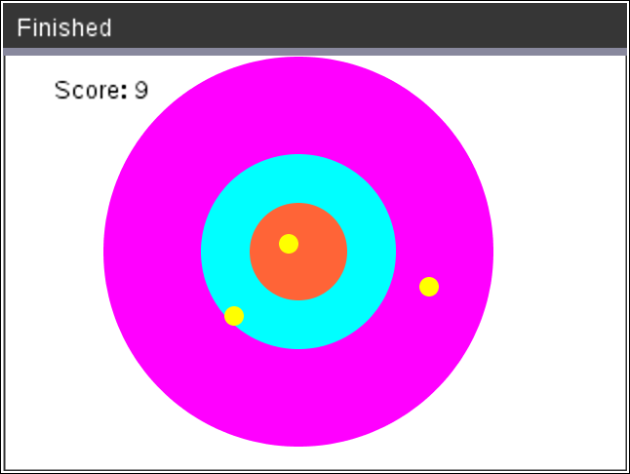•• ##### Device
•TI-Nspire™ CX II
•TI-Nspire™ CX II CAS
• ##### Software

TI-Nspire™ CX

5.2

# Explorations with Coding: Factor Darts

by Texas Instruments#### Objectives

• Use the randint() function to generate random integers.
• Use for loops to repeat code.
• Use selection statements to make decisions.
• Use the draw functions from the TI-Draw library to create circles and text.
• Use the sleep function from the Time library to create animation.
• Practice solving quadratic equations
• Use the distance formula to find the distance between two points
• Use the modulus operator, %, to find a remainder

#### About the Lesson

In this lesson, students will create a dart game that uses factoring to earn darts.  The game will generate 4 random factor problems.  If students answer correctly, they will earn a dart.  Answer two questions in a row, earn an additional dart.  After factoring 4 questions, toss all the darts to earn a score.  How high can you score?  Answer all 4 questions correctly, earn 6 darts.  Can you score a perfect 30?

Note: This lesson requires the use of TI-Nspire™ CX II technology with OS 5.2 and above.